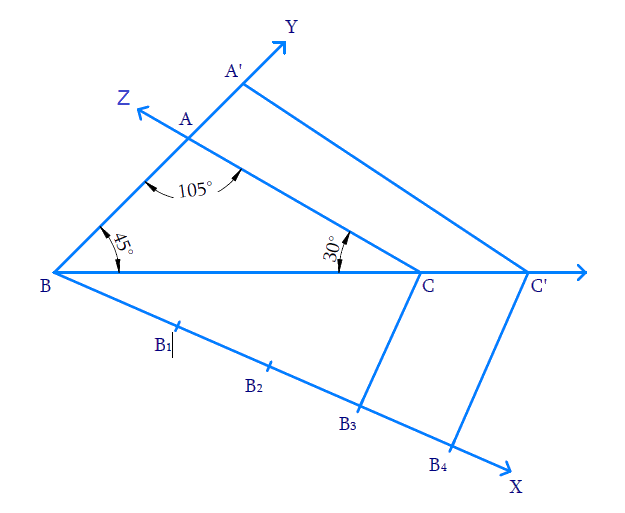# Ex.11.1 Q6 Constructions Solution - NCERT Maths Class 10

Go back to  'Ex.11.1'

## Question

Draw a triangle ABC with side \begin{align}{{BC }} = {{ }}7\,{\rm{ cm,}}\,\,\angle {{B }} = {{ }}45^\circ ,\,\,\angle {{A }} = {{ }}105^\circ .\end{align} Then, construct a triangle whose sides are \begin{align}\frac{4}{3}\end{align} times the corresponding sides of \begin{align}\Delta{ ABC}\end{align}.

Video Solution
Constructions
Ex 11.1 | Question 6

## Text Solution

#### What is known?

One side and $$2$$ angles of a triangle and the ratio of corresponding sides of $$2$$ triangles.

Construction.

#### Reasoning:

• Draw the triangle with the given conditions.
• Then draw another line which makes an acute angle with the base line. Divide the line into $$m + n$$ parts where $$m$$ and $$n$$ are the ratio given.
• Two triangles are said to be similar if their corresponding angles are equal. They are said to satisfy Angle-Angle-Angle (AAA) Axiom.
• Basic proportionality theorem states that, “If a straight line is drawn parallel to a side of a triangle, then it divides the other two sides proportionally".

#### Steps:Steps of construction:

(i) Draw $${{BC = 7}}\,{\rm{cm}}{{.}}$$ At $$B,$$ make an angle $$\angle {{CBY}} = {45^ \circ }$$ and at $$C,$$ make $$\angle {{BCZ}} = 30^\circ \,\,\left[ {{{180}^ \circ } - \left( {{{45}^ \circ } + {{105}^ \circ }} \right)} \right]$$ . Both $$BY$$ and $$CZ$$ intersect at $$A$$ and thus $${{\Delta ABC}}$$ is constructed.

(ii) Draw the ray $$BX$$ so that $$\angle {{CBX}}$$ is acute.

(iii) Mark $$4$$ \begin{align}\left( {4{{ }} > {{ }}3\,{\rm{ in}}\,\,\frac{4}{3}} \right)\end{align} points $${{{B}}_{{1}}}{{,}}{{{B}}_{{2}}}{{,}}{{{B}}_{{3}}}{{,}}{{{B}}_{{4}}}$$ on BX such that $${{B}}{{{B}}_{{1}}}{{ = }}{{{B}}_{{1}}}{{{B}}_{{2}}}{{ = }}{{{B}}_{{2}}}{{{B}}_{{3}}}{{ = }}{{{B}}_{{3}}}{{{B}}_{{4}}}$$

(iv) Join $$B_3$$ (third point on $$BX,\;3\, < 4$$ in \begin{align}\frac{4}{3}\end{align}) to $$C$$ and draw $${{{B}}_{{4}}}{{C'}}$$ parallel to $$BC$$ such that $$C’$$ lies on the extension of $$BC.$$

(v) Draw $$C’A’$$ parallel to $$CA$$ to intersect the extension of $$BA$$ at $$A’.$$

Now, $$\Delta {{A'BC'}}$$ is the required triangle similar to $${{\Delta ABC}}$$ where,

\begin{align}\frac{{{{BA'}}}}{{{{BA}}}}{{ = }}\frac{{{{BC'}}}}{{{{BC}}}}{{ = }}\frac{{{{C'A'}}}}{{{{CA}}}}{{ = }}\frac{{{4}}}{{{3}}}\end{align}

Proof:

In $${{\Delta B}}{{{B}}_{{4}}}{{C',}}\,\,{{{B}}_{{3}}}{{C}}\,\,{{||}}\,\,{{{B}}_{{4}}}{{C'}}$$

Hence by Basic proportionality theorem,

\begin{align}\frac{{{{{B}}_{{3}}}{{{B}}_{{4}}}}}{{{{B}}{{{B}}_{{3}}}}}&= \frac{{{{CC'}}}}{{{{BC}}}}{{ = }}\frac{{{1}}}{{{3}}}\\\frac{{{{CC'}}}}{{{{BC}}}}{{ + 1 }}&=\frac{{{1}}}{{{3}}}{{ + 1}}\,\quad{\rm{(Adding}}\,{{1)}}\\\frac{{{{BC + CC'}}}}{{{{BC}}}}& = \frac{{{4}}}{{{3}}}\\\frac{{{{BC'}}}}{{{{BC}}}}& = \frac{{{4}}}{{{3}}}\end{align}

Consider $$\Delta BA'C'$$ and  $$\Delta BAC$$

$$\angle {{A'BC'}}\, = \angle {{ABC}} = 45^\circ$$

$$\angle {{BC'A'}} = \angle {{BCA}} = 30^\circ$$ (Correspomding angles as $$\,\,{{CA}}\,{{||}}\,\,{{C'A'}}$$ )

$$\angle {{BA'C'}} = \angle {{BAC}} = 105^\circ$$ (Corresponding angles )

By AAA axiom, $${{\Delta BA'C' \sim \Delta BAC}}$$

Hence corresponding sides are proportional

\begin{align}\frac{{{{BA'}}}}{{{{BA}}}}{{ = }}\frac{{{{BC'}}}}{{{{BC}}}}{{ = }}\frac{{{{C'A'}}}}{{{{CA}}}}{{ = }}\frac{{{4}}}{{{3}}}\end{align}

Learn from the best math teachers and top your exams

• Live one on one classroom and doubt clearing
• Practice worksheets in and after class for conceptual clarity
• Personalized curriculum to keep up with school